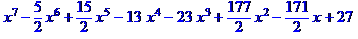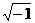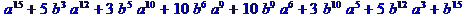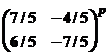WORCESTER POLYTECHNIC INSTITUTE

TWENTIETH ANNUAL INVITATIONAL MATH MEET

OCTOBER 17, 2007

TEAM EXAM QUESTION SHEET WITH ANSWERS

1. A ball was floating in a lake when the lake froze. The ball was removed (without breaking the ice), leaving a hole 24 cm across the top and 8 cm deep. What was the radius of the ball in centimeters?

Ans:  13 cm

1. Point of tangency of two spheres described by

x2 + y2 + z2 = 121

(x-4)2 + (y-12)2 + (z-18)2  =  121Ans:  (2,6,9)

1. Consider the graphs of   y = Ax2  and  y2 + 3 = x2 + 4y,  where A is a positive constant and x  and  y  are real variables.  In how many points do the two graphs intersect?

Ans:  2

1. A certain 5 digit number has the property that if a 1 is placed after it, it is 3 times as large as with a 1 placed before it. What is that number?

Ans:  42857

1. We have two concentric circles and wish to find the area of the annulus between them.

If we draw a chord through the outer circle tangent to the inner circle, its length is 20 inches. What is that area?

Ans:  100Pi

1. Factor the following polynomial over the reals as completely as possible:Ans:  (x-3/2)(x+2)(x2 + 9)(x-1)3

7.    If   z =  2 +√2  i,    what is z20 ??          (where   i =)

Ans:  z = -1048576

8.   Simplify  (a + b)15  mod 15

ans:1. Simplify   3218  mod 7.

Ans:  2

1.  Determine the file size in Megabytes (Mb) for a digital recording made with samples of

size 2 bytes taken 44,100 times per second, in stereo, for 40 minutes. Your answer

should be rounded to the nearest tenth of a Mb.

Ans:  423.4 Mb

11.   Find the sum  17 + 22 + 27+. . . +182

Ans:  3383

12.   If it is given that

logx w=24    logy w =40     logxyz w = 12

then what is  logzw ?     (x, y and z are all positive numbers)

Ans:  60

13.   Simplify the following to a single fraction

-5/4 + 5/8 – 5/16 + 5/32 . . .   - 5/1024

Ans:  -855/1024

14.   Determinewhere p is prime and positive.

Ans:  same matrix

(true for odd powers therefore prime)